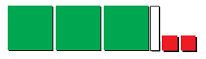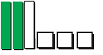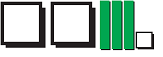# Characteristics of polynomials

### Characteristics of polynomials

A polynomial consists of constants, variables and exponents. Polynomials are named based on the number of terms they have. Polynomials with 1 term are called monomials. Those with 2 terms are called binomials, and the ones with 3 terms are called trinomials.

#### Lessons

• Introduction
a)
What is a polynomial?
• Review on Variables, Coefficients, and Expressions
• What are Monomials, Binomials, and Trinomials?
• What are the Degree, Leading Term, and Constant term of a polynomial?
• Name polynomials based on degree: Quadratic, Cubic, Quartic, Quintic, etc.

b)
How to find the degree of a polynomial?

• 1.
Determine the number of terms and the name for each of the expression.
a)
$- 5{y^2}$

b)
$2{x^2} + 4x + 6$

c)
$- 7.3$

d)
$y^3 + ay + nx - x^2$

• 2.
Find the degree and number of terms for each of the following expression.
a)
$2xy - 3{y^2} + 6$

b)
$4{a^3} + a$

c)
$- 4$

• 3.
Write the expression for each set of the algebra tiles.
a)b)c)• 4.
Peter wants to buy some comic books and magazines. A bookstore sells comic books for $12 each and magazines for$8 each.
a)
Write an expression to represent the money Peter needs to spend on both comic books and magazines.

b)
How much does Peter need to spend if he wants 9 comic books and 3 magazines?

c)
Write a new expression for Peter's expenditure on books if the bookstore raises the price of a comic book to $15 and a magazine to$10.